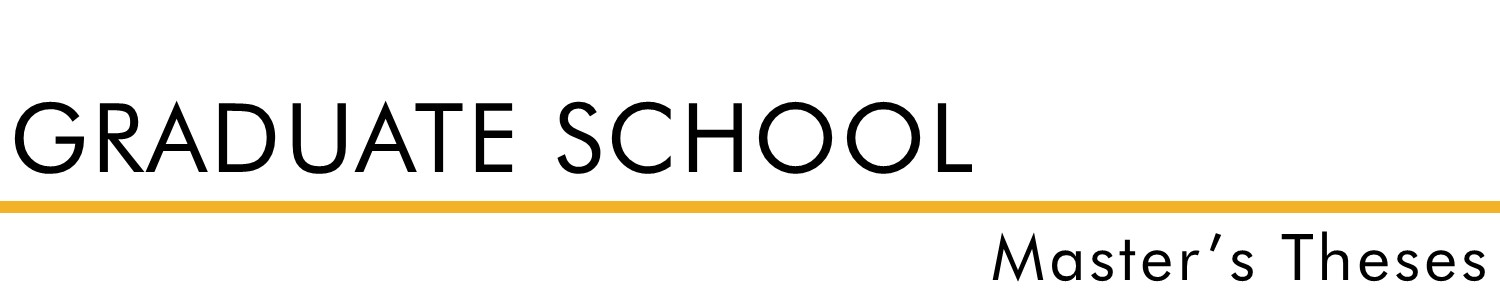Thesis

Fall 1937

#### Degree Name

Master of Science (MS)

Math

Dr. E.E. Colyer

#### Abstract

Any student of mathematics is familiar with the importance of the process of integration. Integration is as fundamental to analysis as the basic principles of the number theory are to arithmetical calculation. Some of the common applications of integration are, finding the distance a falling body has travelled during a particular interval of time; to determine the equation of a curve, given different conditions (such as slope of the curve equal numerically to one-half the abscissa, or some similar problem); motion of a projectile; motion in a resisting medium; finding areas and volumes of revolution; length of a curve; areas of surfaces of revolution; work of expanding gases; and numerous other practical uses. With these many useful applications in mind, the author chose the problem of studying the various methods of integration. It is his earnest desire to learn more about the theory of this interesting subject and to summarize briefly the more familiar definitions of the Riemann and Lebesgue integrals and then to consider less familiar modifications of these definitions. Because of the wealth of material on these subjects, it will be necessary to reduce the discussion of each integral to a minimum. Several important existence theorems will be proven for the Riemann and Lebesgue integrals; followed by a comparison of these two definitions. In discussing the modifications of the above definitions, the author will show the difference between the modification and previous definitions . Lastly, he will offer the opinions of several outstanding mathematicians of the present time regarding the possible trend of integration in the future. In examining the abstracts of theses available in this library and that of the University of Kansas, the author found only one thesis previously written on integration, and that was a Doctor's thesis written on the “Stieltjes integral.”

#### Comments

Notice: This material may be protected by copyright law (Title 17 U.S. Code).

#### Rights

Copyright 1937 Donald Golden

COinS